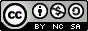# Universidad de Zaragoza Repository

 TAZ-TFM-2010-037

Numerical simulation of one-dimensional transient vertical flow in variably saturated soils

Caviedes Voullieme, Daniel Eduardo
García Navarro, Pilar (dir.)

Universidad de Zaragoza, CPS, 2010
Ciencia y Tecnología de Materiales y Fluidos department, Mecánica de Fluidos area

Máster Universitario en Mecánica Aplicada

Abstract: Water flow in variably saturated (saturated/unsaturated) soils is commonly modeled by means of Richards' equation. This equation has no general analytical solution and the use of numerical approximations is necessary. It can be presented in three physically equivalent forms which are based on different variables and show different mathematical properties. In this work, these forms are derived from the general mathematical model and analyzed from a numerical perspective in order to understand the interactions between the differential equations and the numerical methods required in each case. The goals of this work are, on the one hand, to describe the physical and mathematical reasoning which leads to the formulation of the general mathemati- cal model of flow in porous media, the discussion of the concepts and assumptions which allow to develop Richards' equation, and on the other hand, to establish the properties and limitations of several numerical schemes to approximate the solutions of flows in variably saturated soils. The approach for the mathematical model is to average a microscopic, single- phase flow equation into a macroscopic scale which allows to describe porous media in a practical way, and to consider the necessary assumptions to state Richards' equation as a particular flow case. The porous media constitutive model completes the mathematical model. In this work the Mualem-van Genuchten model and some variants are included. For the numerical model, several schemes are developed for the 1D Richards' equation in the vertical direction. Explicit and implicit centered finite difference schemes are used in this work. The key numerical aspects of interest are those of mass conservation, stability and efficiency. Another key aspect, which is not only numerical is that of continuity from unsaturated into saturated regimes. The constitutive models affect the numerical schemes and some issues arise because of the high non-linearity of the functions, in particular the hydraulic conductivity function. Appropriate discretization of hydraulic conductivity for estimation of flux between numerical cells is a sensible issue wich has been studied by many authors and is treated in this work. All of these issues are analyzed individually and as interrelated problems in the schemes. Validation and test cases are presented and the response of the model to different problems and parameters is examined. From them, it is concluded that the explicit and the implicit schemes based on the mixed form of Richards' equation are better suited for unsaturated problems. For variably saturated problems, the implicit scheme based on the mixed form is the best choice, since the explicit model cannot solve saturation conditions. Conditional stability of the explicit model affects negatively its performance in certain cases, which also leads to the conclusion that the implicit scheme is more efficient and realiable.

Free keyword(s): Richards' equation ; Unsaturated soils ; Porous media ; Finite differences ; Partial differential equations
Tipo de Trabajo Académico: Trabajo Fin de Master
Notas: Resumen disponible también en españolEl registro pertenece a las siguientes colecciones:
Academic Works > Trabajos Académicos por Centro > centro-politecnico-superior
Academic Works > End-of-master works

Rate this document:

1
2
3

(Not yet reviewed)